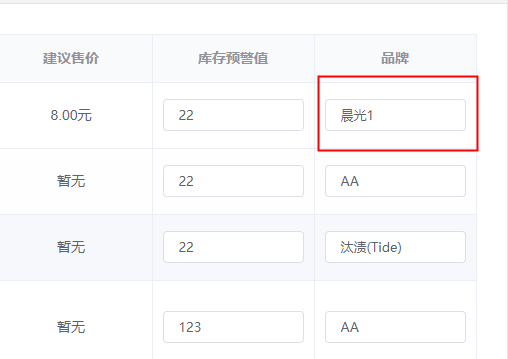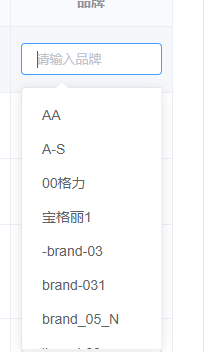# Vue中使用jqueryjquery中的触发事件可以自己定义在mounted中，如果需要使用vue中data的数据，直接使用this.msg是无法使用的，需要另外定义const _this = this,`const _this = this \$('body').delegate('.el-input__inner', 'focus', function(e) { // 因为是动态生成的，所以选择这种方式来监听事件if (e.target.placeholder === '请输入品牌') { // 当聚焦在品牌输入框时触发  \$(this).addClass('inputList') // 加上一个类名inputList  if (\$('.inputList').length === 2) { // 判断下一次点击的位置，当从第一个输入框改值后下一个聚焦位置还是品牌下的输入框时 if (!\$('.inputList').not(this).val()) {\$('.inputList').not(this).css('border', '1px solid #67c23a') } else {let num = 0for (let j = 0; j < _this.goodsBrands.length; j  ) {  if (\$('.inputList').not(this).val() === _this.goodsBrands[j].value) { num  = 1 break  }}if (num === 0) {  _this.\$message.error('输入的品牌不在品牌列表中')  \$('.inputList').not(this).css('border', '1px solid #F56C6C')} else {  \$('.inputList').not(this).css('border', '1px solid #67c23a')} }  }  \$('.el-input__inner').not(this).removeClass('inputList') // 逻辑执行完之后删除当前聚焦位置以外的inputList类，这样就保持.inputList长度最多为2} })`
`此处是为了模拟出输入框的change事件，因为在聚焦时会添加inputList这个类名。所以这里可以判断是否是自己想要的位置，如果是的话给一个标志位getindex,当点击到品牌下的输入框时，如果第二次的点击位置不是品牌其他位置的输入框，则进行逻辑判断`
` \$(document).click(function(e) { // focus触发时也会触发这个事件，并且触发在focus事件之后if (e.target.classList === 'inputList') { // 判断是否点击在对应位置  _this.getIndex = 1  // 用于标志在聚焦事件触发后的下一次点击是否同样会触发聚焦，如果是则把第二次点击当成第一次重新判断，等到下一次点击再来判断} else {  if (_this.getIndex === 1) {  if (!\$('.inputList').eq(0).val()) {\$('.inputList').eq(0).css('border', '1px solid #67c23a') } else {let num = 0for (let j = 0; j < _this.goodsBrands.length; j  ) {  if (\$('.inputList').eq(0).val() === _this.goodsBrands[j].value) { num  = 1 break  }}if (num === 0) {  _this.\$message.error('输入的品牌不在品牌列表中')  \$('.inputList').eq(0).css('border', '1px solid #F56C6C')} else {  \$('.inputList').eq(0).css('border', '1px solid #67c23a')} } \$('.el-input__inner').removeClass('inputList') _this.getIndex = 0 // 每次第二次点击了其他位置时，getIndex重新计数  }} })`